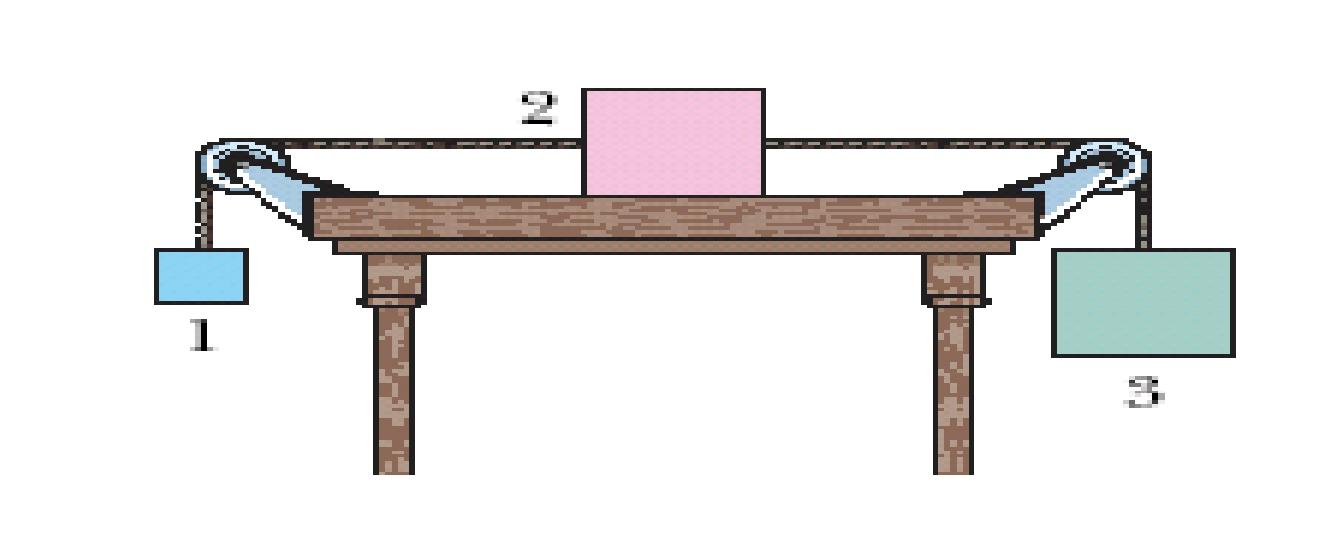# A classical mechanics problem by Ken OsakoWhen the three blocks in the figure are released from rest, they accelerate with a magnitude of $1 \text{ m/s}^2$. Block 1 has mass $M$, block 2 has $2M$, and block 3 has $2M$. What is the coefficient of kinetic friction between block 2 and the table?

Take $g=9.8 \text{ m/s} ^2$.

×